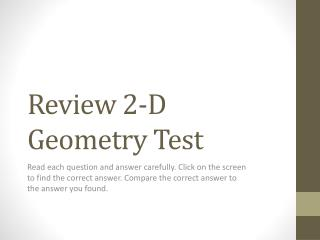DownloadDownload PresentationReview 2-D Geometry Test

# Review 2-D Geometry Test

Télécharger la présentation## Review 2-D Geometry Test

- - - - - - - - - - - - - - - - - - - - - - - - - - - E N D - - - - - - - - - - - - - - - - - - - - - - - - - - -
##### Presentation Transcript

1. Review 2-D Geometry Test Read each question and answer carefully. Click on the screen to find the correct answer. Compare the correct answer to the answer you found.

2. Identify each polygon by its sides. Then classify the polygon as convex or concave. Identify the figure as regular or non-regular. Octagon Concave Non-regular Hexagon Convex Regular

3. Identify the triangles by their angles and their sides Scalene Right Acute Equilateral Isosceles Obtuse

4. Find the measure of each missing angle. 100 100 X 68 37 Y 80 X = 75 Y = 80

5. Find the area and perimeter of each shape. A = 30 in² P = 30 in 5in 3m 4m 12in 7m A = 21 m² P = 22 m 11ft 2.5ft 8ft 7ft 10ft 21ft A= 112ft² P = 48ft A=25ft² P= 25 ft

6. Find the area and circumference of the figure. D= 10m R=5m Area= m² C = = 10m

7. Word Problems • One side of a square is 6 m long. How long is each of the other three sides? • The side of a square is 3 meters long. What is the area? • A label on a 1-gallon can of paint indicates you can cover about 400 square feet of surface. Is that enough paint to cover a rectangular wall that measures 25 feet by 8 feet? • The distance from the center of a circle to the edge of the circle is 2 feet. What is the greatest distance across the circle? 6meters 9 m² Yes. 8 x 25 = 200 4 ft since that is the diameter which is the greatest distance

8. Transformations: List all of the following types of transformations that satisfy each description: reflection, translation, rotation. • a. The transformation is also called a turn. • b. The transformation preserves the orientation of a figure. • c. The transformation is the composite of successive reflections over two intersecting lines. • d. The transformation is the composite of successive reflections over two parallel lines. • e. A specific transformation is defined by a fixed point and a specified angle. • f. The transformation is also called a slide. • g. The transformation is also called a flip. rotation translation rotation translation rotation translation reflection

9. Determine the number of lines of symmetry for each figure described below. Four • a. a square • b. an isosceles triangle (not equilateral) • c. a regular hexagon • d. an isosceles trapezoid • e. the letter E one Six one one

10. Transformation Impact Sam has a rectangular garden plot 25 feet by 50 feet. He plans next year to cut down on the size of the garden. The new dimensions will be changed by a factor of 4/5. Which statement is true about the area of next year’s garden? The area of next year’s garden will be reduced by: • 4/5 • 16/25 • 8/25 • 64/125 B. 16/25

11. Pythagorean Theorem • You place the base of a 10 foot ladder 8 feet from a building. How high does the ladder reach? • 6 ft • 12 ft • 20 ft • 36 ft A. 6ft

12. Pythagorean Theorem Teddy is making a picture frame for his mother. The dimensions of the frame are 18 inches by 10 inches. What should the diagonal measure in order to have each corner be a right angle? • 18 in • 28 in • 20.591 in • 21.345 in C. 20.591 in